# Dynamic Light Scattering (DLS) Microrheology - A Guide

Dynamic Light Scattering (DLS) — occasionally referred to as Quasi-Elastic Light Scattering (QELS) or Photon Correlation Spectroscopy (PCS) — is a method widely used for measuring the size of particles suspended in a liquid, usually in the sub-micrometer regime (1).

In fact, DLS measures the Brownian motion of the particles in the liquid, and the technique is the foundation for embedded probe particle Microrheology techniques.

## The Generalized Stokes-Einstein Relation

For a particle moving freely because of thermal fluctuations in an ideal viscous (Newtonian) fluid, the Mean Square Displacement (MSD) increases linearly with time, where the slope of this increase is given by the diffusion coefficient of the particle (2)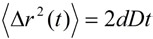(1)

where <Δr2(t)> is the MSD, D is the diffusion coefficient of the particle, and d is the dimensionality of the motion (with d = 2 for motion in a plane and d = 3 for motion in space).

In an ideal viscous (Newtonian) system, the diffusion coefficient can be given as a function of the viscosity of the fluid medium, the size of the particle, and the temperature using the Stokes-Einstein relation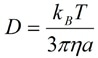(2)

where η is the viscosity of the fluid medium, a is the diameter of the particle, T is the temperature, and kB is the Boltzmann constant. Hence, the Stokes-Einstein relation relates the MSD of the particle to the fluid’s viscosity.

However, for viscoelastic (non-Newtonian) complex fluids — where the medium dissipates energy (viscosity), as well as stores energy (elasticity) — Equation (2) does not describe the complete behavior of the system. As the elasticity of the suspending medium becomes noteworthy, particle motion becomes sub-diffusive and deviations from linearity in the slope of the MSD become clear. The validation of a more general strategy for extending the approach of linking MSD to measure the linear viscoelastic moduli of complex fluids with the help of DLS laid the foundation for modern Microrheology (3).

A generalized Langevin equation is used to describe the thermally driven motion of a particle dispersed in a complex fluid (4, 5)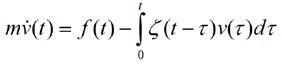(3)

where m is the mass of the particle, v& is the particle acceleration,  f is the total force acting on the particle, and ζ is a time-dependent memory function that accounts for the elasticity in the system. By solving the equation of motion for the case where the only forces on the particle are due to thermal fluctuations in the system, the Generalized Stokes-Einstein Relation (3, 4) is formed, that relates the Mean Square Displacement (MSD) of the tracer to the viscoelastic modulus of the complex fluid medium surrounding the particle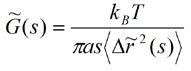(4)

where the tilde symbol represents the Laplace transformation, and s is the Laplace frequency; the remaining variables are the same as those in Equation (2). G* is the viscoelastic modulus of the system as experienced by the probe particle. Equation (4) can be recast in Fourier space by using the identity s= iω to obtain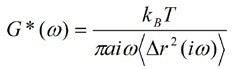(5)

where the angular frequency ω is given by ω = 2π/t.

The Generalized Stokes-Einstein Relation is based on the assumption that the complex fluid medium is a continuum around the particle, that is, the length scales of the microstructures in the complex fluid are small when compared to the size of the particle.

## Extracting Rheological Data from DLS Measurements

A Dynamic Light Scattering (DLS) experiment measures the autocorrelation function (ACF) of the light scattered by particles experiencing thermally driven motion within a material being studied. It can be shown that the ACF, g1(τ), can be given as a function of the Mean Square Displacement (MSD) of the scattering particles (5)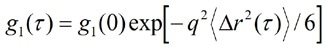(6)

where g1(0) is the value of the autocorrelation function at zero time (or intercept), and q is the scattering vector given by the equation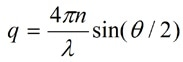(7)

where θ is the scattering angle, n is the refractive index of the medium, and λ is the wavelength of the light. The correlation time τ is related to the time t by τ = 2πt, so that ω = 1/τ.

Thus, a DLS Microrheology experiment demands the addition of probe (tracer) particles of a known size into the medium of interest to measure the ACF of the light scattered by the probe particles. Then, the following relation between the MSD of the probe particles and the ACF of the scattered light is obtained from Equation (6)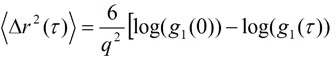(8)

where g1(0) is the estimated intercept of the value of the autocorrelation function and q is the scattering vector as mentioned before. On the whole, a huge value of the intercept (above 0.8) signifies the absence of multiple scattering (scattering from the probe particles is expected to be high in comparison to background scattering from the suspending medium).

By calculating the Fourier transform of the MSD and substituting it in Equation (5), the viscoelastic modulus can be obtained. However, due to the fact that the correlation data is generally logarithmically spaced in time, and does not lend itself to the standard methods of obtaining the Fourier transform, a method based on a power law expansion of the MSD can be employed (4). This method algebraically estimates the complex shear modulus by expanding <Δr2(t)> locally around t = 1/ω to give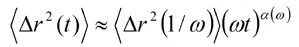(9)

where <Δr2(1/ω)> is the magnitude of <Δr2(t)> at t = 1/ω and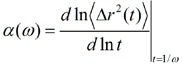(10)

is the power law exponent describing the logarithmic slope of <Δr2(t)> at t = 1/ω.

For thermally driven motion of the probe particles, the slope of the logarithmic time derivative of the MSD will be equal to zero in an elastic medium (completed arrested motion), one in a purely viscous medium (diffusive motion), and will lie between these extremes in a complex viscoelastic fluid medium.

The expressions for the frequency-dependent viscoelastic moduli G′, elastic (storage) modulus and G″, viscous (loss) modulus (4) are given by evaluating the Fourier transform of the power law behavior of the MSD, substituting into Equation (5), and using Euler’s equation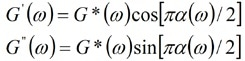(11)

where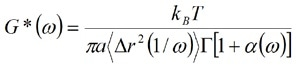(12)

and where Г represents the gamma function which is obtained as a result of the Fourier transform of the power law behavior of the MSD.

Then, complex viscosity, η*, can be calculated by using the following relation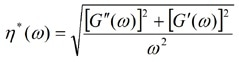(13)

## Applicability of DLS Microrheology

It is necessary to take into account the conditions in which either DLS measurements or the assumptions within the Generalized Stokes-Einstein Relation become unreliable to guarantee robust and consistent DLS Microrheology data.

From the point of view of DLS measurement, it is essential to make sure that the dispersed probe particles are the dominant scatterers in the system, and that the condition of single scattering is maintained. As discussed earlier, specific quality criteria are available that can be applied, for instance, to the autocorrelation function to underline appropriate data quality requirements. Additionally, the use of defined method development procedures is vital to carry out valid DLS Microrheology measurements, for example, to evaluate satisfactory dispersion of probe particles.

The Generalized Stokes-Einstein Relation is the supporting basis of Microrheology techniques, and its general applicability has been proven to hold across a wide range of complex fluid types, allowing quantitative rheological data to be measured over a broad range of frequencies.

However, specific cases have been found where assumptions in the Generalized Stokes-Einstein Relation can fail, and where agreement between bulk rheological measurements and Microrheology data cannot generally be expected (4).

In the case of DLS Microrheology measurements, the following are some of the significant cases where assumptions within the Generalized Stokes-Einstein Relation may be violated, and where agreement with bulk (macro) rheological measurements may not happen:

• Influence of probe particle-matrix interactions

Reducing particle-matrix interactions is a major requirement of a Microrheology experiment because the existence of significant physical (e.g. depletion or electrostatic) or chemical interactions of the embedded probe with the surrounding material can change the local material environment and have an impact on the diffusivity in a measurable way. Guaranteeing a suitable choice of particle surface chemistry is a vital element of method development for DLS Microrheology measurements for a specific complex fluid.

• Influence of probe particle size and material heterogeneity

The probe size used in DLS Microrheology measurements can considerably influence the extracted rheological parameters (7). It is necessary for the probe size to be greater than the relevant microstructural length scale, for example, mesh size in a polymer network, in a way that it probes the bulk response, that is, the assumption of continuum viscoelasticity holds. (It should also be noted that the particle size should not be so large that there is considerable sedimentation on the timescale of the experiment.) Another vital element of method development for DLS Microrheology measurements for a specific complex fluid is guaranteeing an appropriate choice of probe particle size to allow bulk material properties to be extracted.

It is vital to note that the capability of probing local material heterogeneity on a broad range of microstructural length scales with targeted Microrheology techniques enables obtaining new information (not available from bulk measurements), for further new insights into material microstructures and characterization.

• Materials with evolving or aging microstructure

A material can be regarded as quasi-steady state if the timescale of the material structural evolution is gradual in comparison with (experimental) data sampling times. In this case, DLS Microrheology can be employed to monitor material structure evolution.

• Aggregating or gelling systems near to the gel point

Thanks to its sensitivity to probe very soft structures, and find out very low values of viscoelastic modulus (8), DLS Microrheology is an important technique for tracking the onset of aggregation or gelation. Beyond the gel point, the system becomes non-ergodic, and the contribution of the aggregated structures to the overall scattering increases considerably to the point of making single scattering DLS measurements unreliable.

1. Malvern Instruments, Dynamic Light Scattering: An Introduction in 30 Minutes. MRK656-01.
2. Chaikin PM, Lubensky TC, 2000, Principles of condensed matter physics, Cambridge University Press 371.
3. Mason TG, Weitz DA, 1995, Optical measurements of frequency-dependent linear viscoelastic moduli of complex fluids. Phys. Rev. Lett. 74: 1250-1253.
4. Mason TG, 2000, Estimating the viscoelastic moduli of complex fluids using the generalized Stokes-Einstein equation. Rheol. Acta 39: 371-378.
5. Dasgupta BR, Tee S-Y, Crocker JC, Frisken BJ, Weitz DA, 2002, Microrheology of polyethylene oxide using diffusing wave spectroscopy and single scattering. Phys. Rev. E 65:051505.
6. Squires TM, Mason TG, 2010, Fluid Mechanics of Microrheology. Annu. Rev. Fluid Mech. 42: 413-438.
7. Lu Q, Solomon MJ, 2002, Probe size effects on the microrheology of associating polymer solutions. Phys. Rev. E 66:061504.
8. Amin S, Rega CA, Jankevics H, 2012, detection of viscoelasticity in aggregating dilute protein solutions through dynamic light scattering based optical microrheology. Rheol. Acta 51: 329-342.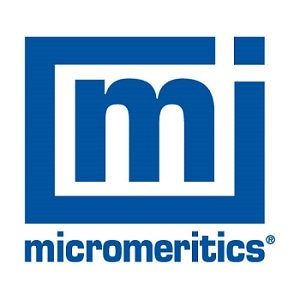This information has been sourced, reviewed and adapted from materials provided by Micromeritics Instrument Corporation.

## Citations

• APA

Micromeritics Instrument Corporation. (2019, December 09). Dynamic Light Scattering (DLS) Microrheology - A Guide. AZoM. Retrieved on October 30, 2020 from https://www.azom.com/article.aspx?ArticleID=16797.

• MLA

Micromeritics Instrument Corporation. "Dynamic Light Scattering (DLS) Microrheology - A Guide". AZoM. 30 October 2020. <https://www.azom.com/article.aspx?ArticleID=16797>.

• Chicago

Micromeritics Instrument Corporation. "Dynamic Light Scattering (DLS) Microrheology - A Guide". AZoM. https://www.azom.com/article.aspx?ArticleID=16797. (accessed October 30, 2020).

• Harvard

Micromeritics Instrument Corporation. 2019. Dynamic Light Scattering (DLS) Microrheology - A Guide. AZoM, viewed 30 October 2020, https://www.azom.com/article.aspx?ArticleID=16797.